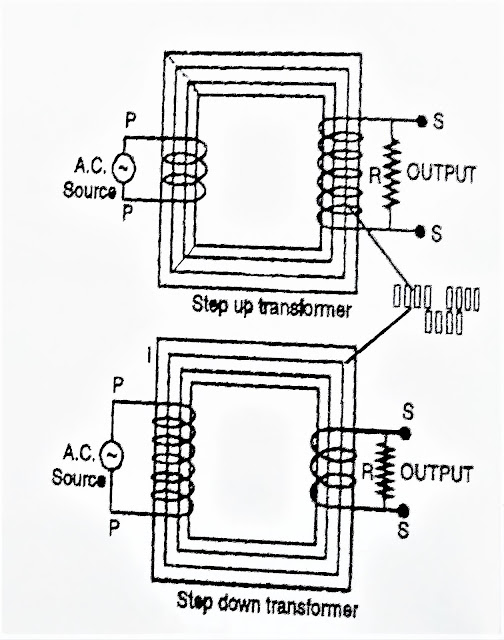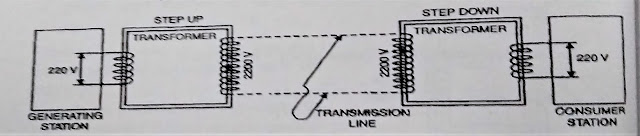## Working, Types and Uses of Transformer - 12 Class Physics NCERT Notes

Get free notes and free pdf file of working, construction, types and uses of electrical transformer for 12 class physics.

## What is a Transformer?

### Principle of an electrical transformer :

It works on the principle of mutual induction. According to which, if two coils are inductively coupled, then an induced e.m.f4 is produced in one coil when a changing current flows through its neighboring coil.

### Construction of electrical transformer :

It consists of two coils P and S wounded over a laminated soft iron care. The coils P and S are called primary and secondary respectively.
The ac input is applied across the Primary and transformed output is obtained across the secondary coil.

### Types of electrical transformer :

Transformers are of two types:

#### Step down transformer :

It converts high alternating voltage at low current into low alternating voltage at high current. In this Np> Ns. Primary is made of thin wire and secondary is made of thick wire.Step-down transformer

Step-up and Step-down transformer :Transformer

#### Theory :

When alternating e.m.f is connected to primary, then due to flow of ac in primary, alternating magnetic flux is linked with the coils. This changing magnetic flux induces an alternating e.m.f. in secondary coil.

Let Np - no. of turns in Primary:
Ns - no. of turns in Secondary
Φp and Φs are magnetic flux linked with Primary and Secondary coils at any instant.

then  Φs ∝ Ns                and      Ep = Np     .................................. (1)
dt
Φp ∝ Np                and       Ep = Np dΦ    ...................................(2)
dt

where Φ is flux linked with, one turn

Φs  = Ns                        and       Es = Ns
Φp     Np                                    Ep    Np

This equation shows that for same power transfer, voltage increases with decrease in current and vice-versa.For ideal transformer, η is 100% but for real transformer, η varies between 90 - 99%.

#### Energy Losses in Transformer :

(i) Flux losses: Coupling between primary and secondary coils is never perfect. So, whole magnetic flux linked with primary gets never linked up with the secondary coil.
(ii) Copper loss: Due to resistance of windows of primary & secondary coils some electrical energy is always converted into heat energy.
(iii) Iron losses: The varying magnetic flux produces eddy currents in iron core, which also leads to the loss of energy in the form of heat. To minimize eddy currents or loss due to eddy currents laminated core is used.

(iv) Hysteresis losses: The alternating currents flowing through the coils magnetizes step up and demagnetizes the iron core again and again over complete cycles. During each cycle of magnetization and demagnetization some energy is lost due to hysteresis and is equal to area of hysteresis loop. This loss can be minimized by selecting a material of core, which has a narrow hysteresis loop.

(v) Humming loss : Due to passage of ac the core of transformer starts vibrating and produces humming sound. So small amount of electrical energy is wasted in the form of humming sound.

### Uses of Transformer:

(i) When electric power is transmitted through the transmission wires, due to resistance of wires, electrical energy is wasted in the form of heat energy. This heat produced is proportional to the square of current flowing & H ∝ I2Rt.
So, when transmission of ac is made at low current and high voltage, using a step-up transformer, loss of electric energy is much reduced.
So by using transformer electric power can be transmitted from one station to another at very high voltage and low current without any significant loss.transformer
(ii) using transformer cost of transmission can be reduced : If transmission is done at high voltage, the line wires required are of low capacity, i.e. thin wires and light poles may be used, due to which cost of transmission will be less.

(iii) A step-down transformer is used to obtain large current for electric welding.

(iv) Step-down transformer is used in induction furnace for melting metals.

(v) Transformers are used in voltage regulators and stabilizers.

(vi) Small transformers are used in radio sets, TV., telephone & loud speakers etc.